# Weakly infinite-dimensional space

(diff) ← Older revision | Latest revision (diff) | Newer revision → (diff)
A topological spacesuch that for any infinite system of pairs of closed subsets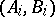of it,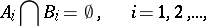there are partitions (cf. Partition)(between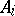and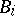) such that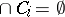. An infinite-dimensional space which is not weakly infinite dimensional is called strongly infinite dimensional. Weakly infinite-dimensional spaces are also called-weakly infinite dimensional. If in the above definition it is further required that some finite subfamily of the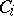's have empty intersection, one obtains the concept of an-weakly infinite-dimensional space.